## research papers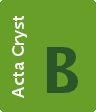STRUCTURAL SCIENCECRYSTAL ENGINEERINGMATERIALS
ISSN: 2052-5206

## A complicated quasicrystal approximant ∊16 predicted by the strong-reflections approach

aStructural Chemistry, Stockholm University, SE-106 91 Stockholm, Sweden, and bInstitut für Festkörperforschung, Forschungszentrum Jülich, D-52425 Jülich, Germany
*Correspondence e-mail: junliang.sun@mmk.su.se, sven.hovmoller@mmk.su.se

(Received 30 July 2009; accepted 14 December 2009)

The structure of a complicated quasicrystal approximant 16 was predicted from a known and related quasicrystal approximant 6 by the strong-reflections approach. Electron-diffraction studies show that in reciprocal space, the positions of the strongest reflections and their intensity distributions are similar for both approximants. By applying the strong-reflections approach, the structure factors of 16 were deduced from those of the known 6 structure. Owing to the different space groups of the two structures, a shift of the phase origin had to be applied in order to obtain the phases of 16. An electron-density map of 16 was calculated by inverse Fourier transformation of the structure factors of the 256 strongest reflections. Similar to that of 6, the predicted structure of 16 contains eight layers in each unit cell, stacked along the b axis. Along the b axis, 16 is built by banana-shaped tiles and pentagonal tiles; this structure is confirmed by high-resolution transmission electron microscopy (HRTEM). The simulated precession electron-diffraction (PED) patterns from the structure model are in good agreement with the experimental ones. 16 with 153 unique atoms in the unit cell is the most complicated approximant structure ever solved or predicted.

### 1. Introduction

The question regarding the exact atomic positions in quasicrystals has confused crystallographers for more than two decades since the discovery of the icosahedral quasicrystal in rapidly solidified Al–Mn alloys (Shechtman et al., 1984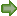). Although quasicrystals are well ordered on the atomic scale, they are not periodic. Furthermore, the presence of defects has made it very difficult to solve their structures by single-crystal X-ray diffraction. An effective way to study the structure of quasicrystals is to start from quasicrystal approximants which often coexist with quasicrystals. This is because the latter normally have similar local atomic structures as quasicrystals. A series of quasicrystal approximants are often closely related in terms of their structures. Until today, many quasicrystal approximants have been found, but only a few of these structures have been solved by X-ray crystallography. One of the examples is the family of approximants in the Al–TM (TM = transition metal) alloys (Klein et al., 1996; Sun & Hiraga, 1996; Balanetskyy, Grushko, Velikanova & Urban, 2004), where the lattice parameters a (≃ 23.5 Å) and b (≃ 16.8 Å) are essentially the same, while the c parameters of the regular structures are ≃12.3, 32.4, 44.9 or 57.0 Å. They are designated as 6, 16, 22, 28… (Balanetskyy, Grushko, Velikanova & Urban, 2004), where the subscript is the index l of the strong (00l) reflection corresponding to the interplanar spacing of ∼ 0.2 nm. Earlier investigations of Al–TM alloys revealed that it was difficult to synthesize Al–TM samples with only a single type of phase. The crystals often consist of different transitional states that are aperiodic in one direction. Owing to the difficulty in growing large ordered single crystals and the complexity of their structures, most of the approximant structures remain unsolved. Indeed, only the 6 structure (also known as ξ′) was solved by single-crystal X-ray diffraction (Boudard et al., 1996). This situation has significantly slowed down the further investigations of quasicrystal structures.

The structures of crystals too small for single-crystal X-ray diffraction can be determined by electron crystallography. Electrostatic potential maps can be obtained by combining the structure-factor phases from HRTEM images with amplitudes from HRTEM images or electron-diffraction patterns. A successful application is to the complicated quasicrystal approximant ν-AlCrFe with space group P63/m, a = 40.687 and c = 12.546 Å (Zou et al., 2003). A three-dimensional electrostatic potential map was calculated by combining the structure-factor phases from HRTEM images and amplitudes from selected-area electron-diffraction (SAED) patterns of 13 zone axes. 124 of the 129 unique atoms in the unit cell were found from the electrostatic potential map. However, this method requires extensive experimental work for the determination of complicated structures such as quasicrystal approximants. First, a sufficient number of high quality images which contain phase information needs to be collected. Unfortunately, the phases from HRTEM are affected by many uncertain factors, such as defocus, astigmatism, crystal thickness and orientation. Extracting the real phases therefore relies on advanced image processing that takes into account these experimental conditions (Klug, 1978–1979; Hovmöller et al., 1984; Zou et al., 1996). Secondly, in order to obtain enough intensity information, many electron-diffraction patterns of different zone axes must be taken. However, as with HRTEM images, high quality kinematic electron-diffraction data are very difficult to obtain, owing to the multiple scattering of electrons (Zou & Hovmöller, 2008).

Another effective way of determining approximant structures, namely the strong-reflections approach, was proposed by some of the present authors (Zou & Hovmöller, 2008; Christensen et al., 2004). This approach is based on the fact that the strongest reflections largely determine the atomic positions in a structure. By analyzing the relationship of the structure-factor amplitudes and phases of reflections from a series of quasicrystal approximants, we found that the strong reflections that are close to each other in reciprocal space have similar structure-factor amplitudes and phases for all the approximants in the series (Zhang, Zou et al., 2006). Therefore, the structure-factor amplitudes and phases of strong reflections for an unknown approximant can be estimated from those of a known related approximant. Atomic positions in the unknown approximant are then obtained directly from the three-dimensional electron-density map calculated by inverse Fourier transformation of the structure-factor amplitudes and phases of the strong reflections. It is important to find the orientation matrix that relates the known and the unknown approximants, and re-index the reflections from the known approximant to the unknown approximant.

Structural models of five compounds in three groups of approximants (see Table 1) have been successfully deduced from their known related structures by the strong-reflections approach (Christensen et al., 2004; Zhang, Zou et al., 2006; Zhang, He et al., 2006; He et al., 2007). For all these five cases listed in Table 1, the phase origins (defined by the space groups) are the same for all structures in the same group. The structure-factor phases of the known approximant are therefore the same as those of the unknown approximant. Here we present the structure of the 16 approximant in an Al–Rh alloy, deduced by the strong-reflections approach from the known structure of the 6 approximant in the same Al–Rh system. The structure determination in the present case is more complicated since the origins in space groups Pnma (known structure 6) and B2mm (unknown structure 16) are different. The structure-factor phase relations of the symmetry-related reflections are not consistent in the two structures. In order to utilize their structure-factor phases, a non-standard origin was first used and then shifted to the final origin for 16. The 16 approximant has the most complicated structure ever predicted/determined by electron crystallography or the strong-reflections approach. Details are presented below.

 Table 1List of quasicrystal approximants solved by the strong-reflections approach
Known structure   Deduced structure
Lattice parameters (Å)       Lattice parameters (Å)
Phase Space group a (Å) b (Å) c (Å) β (°)   Phase Space group a (Å) b (Å) c (Å) β (°) Ref.
m-Al13Co4 C2/m 15.2 8.1 12.4 108   τ2-Al13Co4 P2/m 39.9 8.1 32.2 108 Christensen et al. (2004)
μ3-ZnMgRE P63/mmc 14.6   8.6μ5-ZnMgRE P63/mmc 23.5   8.6   Zhang, Zou et al. (2006)
μ7-ZnMgRE P63/mmc 33.6   8.9
λ-Al4Mn P63/m 28.38   12.39τ(μ)-AlCrSi P63/mmc 32.3   12.4   Zhang, He et al. (2006)
μ′-AlCrSi P63/mmc 20.1   12.4
6 Pnma 23.5 16.8 12.3     16 B2mm 23.5 16.8 32.4   This work

### 2. Experimental

Sample alloys with nominal composition Al75Rh25 and Al77Rh23 were prepared according to the Al–Rh phase diagram (Grushko et al., 2000). In order to obtain 6 and 16, additional annealing was applied for up to 69 h at 1323 K to Al75Rh25 and for 2 h at 1369 K to Al77Rh23. Samples for transmission electron microscopy observation were crushed and dispersed on holey carbon films on Cu grids. Selected-area electron-diffraction (SAED) and precession electron-diffraction (PED) patterns were recorded in a Jeol 2000FX electron microscope operated at 200 kV. PED patterns of 6 and 16 were taken with a precession angle of ∼ 1.1°, using the Spinning Star Precession Unit (Avilov et al., 2007). The 16-bit digitized SAED and PED patterns were analysed by the program ELD (Zou et al., 1993). HRTEM images were recorded on a Jeol 3010 electron microscope with a point resolution of 0.17 nm operated at 300 kV. The projection symmetry of the crystal was determined from the HRTEM images by crystallographic image processing using the program CRISP (Hovmöller, 1992). The program eMap (Oleynikov, 2006) was used for calculating structure factors from the structure model, calculating three-dimensional electron-density maps from crystal structure factors, determining peak positions from the electron-density maps and simulating HRTEM images and precession electron-diffraction patterns from the structure model.

### 3. Results and discussion

#### 3.1. Unit-cell and space-group determination

There are several ways to identify crystallographic features from electron microscopy. For example, by comparing the net of reflections between ZOLZ (zero-order Laue zone) and FOLZ (first-order Laue zone) that emerge at precession angles > 0.5°, the Bravais lattice and glide planes can be inferred (Morniroli et al., 2007). The point group may also be deduced from convergent-beam electron-diffraction (CBED) patterns. Here we determine the space group of 16 by combining SAED and HRTEM techniques. Four electron-diffraction patterns of 16 along the , ,  and  directions are shown in Fig. 1. From these, the unit cell was determined as B-centered orthorhombic with a = 23.5, b = 16.8 and c = 32.4 Å. 16 has almost the same a and b parameters as 6 (unit-cell parameters a = 23.5, b = 16.8, c = 12.3 Å), but c is τ2 times that of 6 (τ ≃ 1.618 is the golden ratio).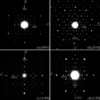Figure 1 Selected-area electron-diffraction patterns of ∊16 along the (a) , (b) , (c)  and (d)  directions.

The reflection conditions of 16 deduced from the SAED patterns are hkl: h + l = 2n.

According to the International Tables for Crystallography (Hahn, 2002), four space groups – B222 (No. 21), Bm2m (No. 35), B2mm (No. 38) and Bmmm (No. 65) – fulfill these reflection conditions. These four space groups are possible for 16 and cannot be distinguished by the reflection conditions only. Fortunately, the projection symmetries along the b axis are different for these space groups: cm for B2mm and cmm for the others. It is possible to determine the projection symmetry of 16 by analyzing the phases extracted from the Fourier transform of the HRTEM images taken along the b axis. This was performed by the program CRISP (Hovmöller, 1992). The projection symmetry of 16 was determined as cm since the HRTEM image gave a much lower average phase error for cm (phase residual = 19.5°) than that for cmm (phase residual = 44.8°). This is also directly confirmed from the HRTEM image taken along  shown in Fig. 2. Only one mirror perpendicular to the c axis appears in the  image, and no mirror perpendicular to the a axis was found. Thus, the projected symmetry along  was determined as cm but not cmm and the only possible space group for 16 is B2mm.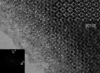Figure 2 HRTEM image of ∊16 taken along the b axis. The Fourier transform of the HRTEM image, a symmetry-imposed image (left) and a simulated image (right) are inserted. There is only one mirror symmetry, perpendicular to the c axis. Unit cells are marked and the banana-shaped tile and the pentagonal tile are outlined in the symmetry-imposed image. The simulation was carrried out with −25 nm defocus and 6.6 nm thickness.

#### 3.2. Deducing a structure model

Once the space group has been determined, we are ready to deduce the structure model of 16 from the structure of 6 using the strong-reflections approach. The most important condition for the strong-reflections approach is that the intensity distribution of the strongest reflections between the known and unknown structures should be similar. Thus, the first step is to identify and relate the corresponding strong reflections of 16 to those of 6 using electron diffraction. For such a purpose, precession electron-diffraction patterns were taken as shown in Fig. 3, since they are less dynamical and show higher resolution (about 0.9 Å) than those of the SAED patterns in Fig. 1. The less-dynamical diffraction intensities obtained by precession electron diffraction make the identification of the corresponding strong reflections easier. As can be seen from the experimental precession electron-diffraction patterns of 6 and 16 in Fig. 3, all the strong reflections in 16 coincide with the strong reflections in 6. Since the a and b parameters are similar for 16 and 6, and the c parameter of 16 (32.4 Å) is about τ2 times that of 6 (12.3 Å, 32.4/12.3 = 2.634), the (hkl) indices of the strong reflections in these two approximants are related by (h k l16) = (h k τ2l6). Here, the golden number τ = (1 + 51/2)/2 is associated with fivefold rotational symmetry of an icosahedral quasicrystal. Elser et al. (Elser & Henley, 1985) used the rational ratio of two successive Fibonacci numbers (1, 1, 2, 3, 5, 8, 13, 21, …, Fn, …; Fn = Fn − 1 + Fn − 2) as an approximation to substitute for the irrational τ to obtain the crystalline approximant of an icosahedral quasicrystal. According to the Fibonacci series, (h k 8) in 16 is related to (h k 3) in 6, (h k 10) in 16 is related to (h k 4) in 6, (h k 26) in 16 is related to (h k 10) in 6, and so on.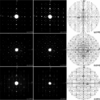Figure 3 Precession electron-diffraction patterns of (a)–(c) ∊6 and (d)–(f) ∊16, and (g)–(i) the simulated precession electron-diffraction patterns from the derived structure model of ∊16. The kinematical simulation conditions are: voltage 200 kV, precession angle 1.1°, and crystal thicknesses (g) 400 Å, (h) 500 Å and (i) 400 Å. The intensity distributions in reciprocal space are similar for ∊6 and ∊16. The corresponding reflections have the same h and k, but l(∊16) ≃ τ2 l(∊6), such that the very strong reflection (006) in ∊6 corresponds to (0016) in ∊16.

To confirm the similarity of the 16 and 6 structures, the R value against the number of corresponding strongest reflections was plotted in Fig. 4without any corrections (such as absorption correction, Lorenz correction and so on). The detailed reflection lists are given as supporting information.1 The reflections from different orientations were merged using their common reflections. It shows that the intensities of the strong corresponding reflections are very close to each other (R = 0.19) for the 30 strongest reflections. As more and more moderately strong reflections are included, the R value increases, reaching 0.29 for the 146 strongest reflections. This kind of R value is close to a typical internal R value for elctron-diffraction data obtained from different particles of the same structure. Thus, we think there is obvious similarity in the ∊16 and ∊6 structures. Note that here we only compared the experimental strong reflections along the three main zone axes, but they constitute a major part of all data. For the 256 strongest reflections (the 45 strongest of which are listed in Table 2), the amplitudes along these three main zone axes sum up to 45% of the total amplitudes in ∊6.

 Table 2List of structure-factor amplitudes and phases of 45 strongest independent reflections of ∊6 (with Pnma) and ∊16 (with P1 and B2mm)
 After the origin has been shifted from that of ∊6 to (0, 0.25, 0.15625), the phases of symmetry-related reflections [Phase ∊16 (P1)] are close to those required by the space group B2mm, with an average phase error of 7.8° and a maximum phase error of 22.5° compared with phases after imposing the B2mm symmetry.
Amplitude Phase               Phase 16 (B2mm)
6 6 and 16 6 (Pnma) and 16 16 Origin shifted (0, 0.025, 0.15625) Symmetry-imposed
h k l   hkl hkl hkl hkl h k l hkl hkl hkl hkl hkl
0 8 0 2815 0 0 0 0 0 8 0 0 0 0 0 0
0 0 10 2338 0 0 0 0 0 0 26 23 23 338 338 0
8 4 3 2279 0 180 180 0 8 4 8 90 90 90 90 90
18 0 3 2262 180 0 0 180 18 0 8 270 270 270 270 270
10 4 0 2259 180 180 180 180 10 4 0 180 180 180 180 180
11 0 8 2211 180 0 0 180 11 0 21 281 281 259 259 270
3 4 5 2205 180 180 180 180 3 4 13 191 191 169 169 180
7 0 5 2036 0 0 0 0 7 0 13 11 11 349 349 0
11 0 2 2021 0 180 180 0 11 0 5 281 281 259 259 270
0 0 6 2018 180 180 180 180 0 0 16 0 0 0 0 0
0 16 0 1567 0 0 0 0 0 16 0 0 0 0 0 0
26 4 0 1471 0 0 0 0 26 4 0 0 0 0 0 0
8 4 13 1471 0 180 180 0 8 4 34 113 113 68 68 90
0 8 10 1457 0 0 0 0 0 8 26 23 23 338 338 0
18 8 3 1454 180 0 0 180 18 8 8 270 270 270 270 270
21 4 8 1433 0 180 180 0 21 4 21 101 101 79 79 90
11 8 8 1406 180 0 0 180 11 8 21 281 281 259 259 270
8 12 3 1288 0 180 180 0 8 12 8 90 90 90 90 90
13 7 5 1275 0 0 180 180 13 7 13 281 281 259 259 270
19 4 5 1269 0 0 0 0 19 4 13 11 11 349 349 0
16 7 0 1269 0 0 180 180 16 7 0 270 270 270 270 270
10 4 10 1256 180 180 180 180 10 4 26 203 203 158 158 180
5 7 8 1241 0 180 0 180 5 7 21 11 11 349 349 0
0 4 0 1234 180 180 180 180 0 4 0 180 180 180 180 180
15 4 8 1216 0 180 180 0 15 4 21 101 101 79 79 90
10 12 0 1194 180 180 180 180 10 12 0 180 180 180 180 180
0 8 6 1181 180 180 180 180 0 8 16 0 0 0 0 0
21 4 2 1178 180 0 0 180 21 4 5 101 101 79 79 90
3 4 11 1169 0 0 0 0 3 4 29 191 191 169 169 180
5 7 2 1163 180 0 180 0 5 7 5 11 11 349 349 0
3 12 5 1162 180 180 180 180 3 12 13 191 191 169 169 180
11 8 2 1151 0 180 180 0 11 8 5 281 281 259 259 270
7 8 5 1126 0 0 0 0 7 8 13 11 11 349 349 0
18 0 7 1057 0 180 180 0 18 0 18 293 293 248 248 270
2 7 3 1038 0 180 0 180 2 7 8 0 0 0 0 0
0 14 0 1035 180 180 180 180 0 14 0 0 0 0 0 0
8 11 3 1010 180 0 180 0 8 11 8 180 180 180 180 180
7 0 11 998 180 180 180 180 7 0 29 11 11 349 349 0
10 0 0 973 0 0 0 0 10 0 0 0 0 0 0 0
15 4 2 970 180 0 0 180 15 4 5 101 101 79 79 90
10 11 0 961 180 180 0 0 10 11 0 90 90 90 90 90
3 11 5 959 180 180 0 0 3 11 13 101 101 79 79 90
22 0 0 955 180 180 180 180 22 0 0 180 180 180 180 180
8 4 7 952 180 0 0 180 8 4 18 113 113 68 68 90
19 12 5 903 0 0 0 0 19 12 13 11 11 349 349 0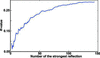Figure 4 R values against the number of corresponding strongest reflections in ∊6 and ∊16. For the 30 strongest reflections, an R value of 0.19 shows good correspondence for the strong reflections in ∊6 and ∊16. As more and more moderately strong reflections are included, the R value increases, reaching 0.29 for the 146 strongest reflections.

The strong reflections in 16 are then deduced from the corresponding reflections in the known 6 according to the relations described above. In order to determine the number of independent strong reflections that are needed to obtain a sufficiently good electron-density map, we first checked the procedure on the 6 structure. Generally speaking, the structure-factor amplitude sum of the strong reflections must be more than 50% of the total amplitude sum in order to generate an electron-density map that represents the structure (Zhang, He et al., 2006). Here we choose the 256 strongest reflections of 6 among the total 2640 independent reflections within 1.0 Å resolution. Table 2lists 45 of them, together with their structure-factor amplitudes and phases. The 256 reflections sum up to 57% of the total amplitude and they were expanded to 1590 reflections according to the symmetry of 6. A three-dimensional electron-density map was calculated from the structure-factor amplitudes and phases of these 1590 reflections by inverse Fourier transformation, using the program eMap (Oleynikov, 2006). All the atomic positions of 6 as determined by single-crystal X-ray diffraction (Boudard et al., 1996) could be found in the three-dimensional density map. This indicates that the 256 strong reflections are sufficient to obtain a correct structure model of 6. Since the strong reflections in 16 coincide with the strong reflections in 6, a reasonable structure model of 16 should be obtained using the 256 strong reflections of 16 deduced from those of 6.

The structure-factor amplitudes of 16 were assigned from those of the corresponding reflections in 6, calculated from the single-crystal X-ray structure model (Table 2). We did not use the amplitudes from the experimental PED data since it was difficult to collect a complete three-dimensional PED data due to mixed phases in the same particle. A new technique, called electron-diffraction tomography, is being developed in our department (Zhang et al., 2010) and may be applied in the future for collecting complete three-dimensional electron-diffraction data. Our earlier studies have shown that the amplitudes taken from the corresponding known approximants are enough to deduce the structure model.

Different from our previous studies (Christensen et al., 2004; Zhang, He et al., 2006; Zhang, Zou et al., 2006; He et al., 2007), the structure-factor phases for the strong reflections of 16 cannot be taken directly from those of 6, since the choice of origin for the space group of 16 (B2mm) is different from that of the space group for 6 (Pnma). Consequently, the phase relations between the symmetry-related reflections in the two space groups are different. For the space group Pnma, the relations are (Table 2):

 (i) If h + l = 2n and k = 2n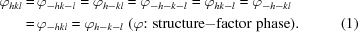(ii) If h + l = 2n and k = 2n + 1: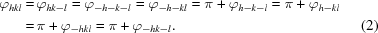(iii) If h + l = 2n + 1 and k = 2n: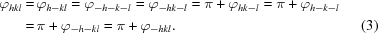(iv) If h + l = 2n + 1 and k = 2n +1: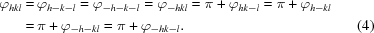For the space group B2mm, the relations are simpler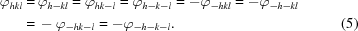One way to overcome the problem of the different phase relationships is to first assume the space group P1 for 16. Starting from 256 strong reflections in 6, they are expanded into 1590 reflections using Pnma symmetry. Based on the strong-reflection approach, each of these strong reflections in 6 structure has one corresponding reflection in the 16 structure in P1 symmetry with the same phases and amplitudes but different indices [see Table 2, column Phase 6 (Pnma) and 16 (P1)]. This 16 structure in P1 symmetry turns out to be very close to B2mm symmetry. Note that although the phase relations between the symmetry-related reflections in the two space groups Pnma and B2mm are different, the phase relations for the strongest reflections in the two structures are almost the same.

The three-dimensional electron-density map of 16 in P1 symmetry gives well resolved peaks that can be assigned to atomic positions, as shown in Fig. 5. From the density map viewed along the b axis (Fig. 5a), the banana-shaped tiles and pentagonal tiles (Balanetskyy, Grushko & Velikanova, 2004) can be identified. The two types of tiles are alternating along the a and c directions and connected to each other. Similar tiles and connections are observed in the  HRTEM image in Fig. 2. The symmetries can be identified from the three-dimensional electron-density map as follows: B-centering, 2 // a, mb, mc, which agrees with the space group B2mm. An origin that is compatible with the space group B2mm was found at a 2mm Wyckoff position, (0, 0.25, 0.15625). Thus, the origin was shifted to this position and the new structure-factor phases were calculated using the following equation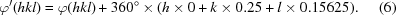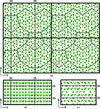Figure 5 A three-dimensional electron-density map of ∊16 calculated from 1590 (256 independent) strong reflections using the space group P1. (a), (b) and (c) are three-dimensional density maps viewed along the b, a and c axes, respectively. Four unit cells are outlined in (a). The symmetry elements can be identified from the density map, with mirrors perpendicular to the b and c axes (marked). The new origin is set on 2mm. The origin shifts obtained from the electron-density maps are Δx = 0, Δy = 0.25 and Δz = 0.15625. Banana-shaped clusters and pentagonal clusters21 are outlined in (a).

The new structure factor phases of 45 reflections from the 256 independent strong reflections are listed in Table 2, together with the symmetry-imposed phases. The average deviation of the phases from the symmetry B2mm is only 7.8°, and the largest phase error is 22.5°. The amplitudes together with the phases of the 256 reflections after imposing the symmetry B2mm were used to calculate a new three-dimensional electron-density map of 16. The three-dimensional density map is very similar to that with P1 symmetry, but the electron densities at symmetry-related positions become exactly identical instead of just similar to each other.

There were 150 unique peaks corresponding to the atomic positions of 16 identified from the three-dimensional density map and the atomic coordinates were determined. There were 33 of them assigned as Rh, and the remaining 117 were Al. The assignment of Rh positions was based on:

 (i) the peak height (the highest peaks) and (ii) chemical knowledge.
Since ∊16 and ∊6 are both members of the same series of icosahedral quasicrystal approximants, they are expected to have very similar local atomic structures. Atoms in similar clusters should have a similar environment, and thus atoms in ∊16 were assigned to form similar clusters as those in ∊6. In addition, three Al atoms were added to complete the structure based on the geometry and similarity to ∊6, see Table 3 in the supplementary material. Most of the atoms have reasonable distances to their neighbors, ranging from 2.2 to 3.1 Å. The final composition of our ∊16 model is Al340Rh99, which fits the synthesis stoichiometry (Al77Rh23) very well.

The precession electron-diffraction patterns simulated using the derived 16 structure model (Figs. 3gi) agree well with the experimental precession electron-diffraction patterns (Figs. 3df). A least-squares refinement was performed with only four refined parameters (overall scale factor, extinction parameters and isotropic atomic displacement parameters for Rh and Al) using the experimental 16 intensities from PED patterns (659 independent reflections within 1.0 Å resolution) in the SHELXL program (Sheldrick, 2008). The refinement converged with R1 = 0.33. Thus, the structure model deduced for 16 from 6 can be taken as a good preliminary model.

#### 3.3. Structure description

The final structure of 16 viewed along the c axis is shown in Fig. 6(a). There are eight layers that are perpendicular to the b axis in each unit cell, including four flat (f) and four puckered (p) layers. Five of the layers, at y = 0 (f1), ∼ 0.125 (p1), ∼ 0.25 (f2), ∼ 0.375 (p2) and 0.5 (f3), are independent (Figs. 6bf). The other three can be generated by a mirror located at y = 0.5. The atoms within the f2 layer deviate slightly from y = 0.25. Since this deviation is very small (< 0.019), f2 is still considered as a flat layer. Therefore, the structure can be described as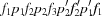, where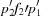are related to the layers p1f2p2 by a mirror operation at y = 0.5. Each layer contains three common basic tilings: a squashed hexagon (h), a pentagonal star (s) and a crown (c) (marked in Fig. 6d). Two h tilings together with one c tiling form a decagonal ring. Each s tiling is surrounded by five inter-connected decagonal rings (marked in Fig. 6f). An additional eight-ring tiling (o) is also found in the layer f2 (marked in Fig. 6e).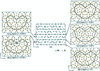Figure 6 The atomic structure of ∊16 (a) viewed along the c axis. Eight layers, including four flat layers (f) and four puckered layers (p), are stacked along the b axis. Five independent layers are identified; at y = 0 (f1), ∼ 0.125 (p1), 0.25 (f2), ∼ 0.375 (p2) and 0.5 (f3). The structure can be described as, where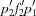are obtained from the layers p1f2p2 by a mirror symmetry at y = 0.5. (b)–(f) The five independent layers viewed along the b axis. Three basic tilings: squashed hexagon (h), pentagonal star (s) and crown (c) are indicated in (d). A decagonal column (Dc) and a pentagonal column (Pc) are marked in (b). A squashed octagon (o) is marked in (e). A pentagonal star (s) surrounded by five hexagonal columns is marked in (f).

The structure of 16 can be described using two types of columns along the b axis: a decagonal column (Dc) and a pentagonal column (Pc) (marked in Fig. 6b). The construction of the decagonal and pentagonal columns is given in Fig. 7. Each puckered layer contributes a decagonal ring to the decagonal column. Between the decagonal rings are small pentagons (in f1 and f3) and irregular tilings (in f2) that are alternating along the b axis (Fig. 7a). The pentagonal column is constructed by pentagonal stars or pentagons that are stacked along the b axis (Fig. 7b). The centers of the decagonal columns are always occupied by the heavy Rh atoms. The decagonal columns are connected to each other through edge-sharing (via two common atoms). Five decagonal columns form a pentagonal tile with a pentagonal column inside (Fig. 6b). Nine decagonal columns form a banana-shaped tile with two pairs of intersecting pentagonal columns inside. The banana-shaped and pentagonal tiles are seen in the HRTEM images (see Fig. 2). Similar decagonal and pentagonal columns have also been observed in the decagonal quasicrystal d-Al–Pd (Li et al., 1996).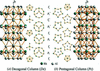Figure 7 Two types of columns related to decagonal quasicrystals. (a) Side view of the decagonal column. Each puckered layer contributes a decagonal ring to the decagonal column. Between the decagonal rings are small pentagons (in f1 and f3) and irregular subunits (in f2) that are alternating along the b axis. (b) Side view of the pentagonal column. The pentagonal column is constructed by pentagonal stars or pentagons that are stacked along the b axis. The centers of the decagonal columns are always occupied by the heavy Rh atoms.

A comparison of the structure features of 6 and 16 is given in Fig. 8. Both structures can be described by the decagonal and pentagonal columns. All decagonal columns in 6 and 16 are connected by edge-sharing. The structure of 6 is constructed by only one type of hexagonal tile built from six decagonal columns with two intersecting pentagonal columns inside. The structure of 16 is constructed by two types of tiles: a banana-shaped tile and a pentagonal tile. The diameter of decagonal and pentagonal columns is about 7.6 Å in both structures. This is also the edge length (marked by thick lines in Fig. 8) of the different tiles. We designate this edge length as S', as the short distance in a Fibonacci series as found in quasicrystal structures. The diagonal distance of the pentagonal tile is τ times longer than S, and thus defined as L'. As shown in Fig. 8, the c parameters can be given by S and L as follows: 6: c = L = τS ≃ 12.3 Å; 16: c = S + L + L ≃ 32.2 Å. Consequently the c parameters for the other approximants in the series are: 22: c = S + L + L + L ≃ 44.5 Å; 28: c = S + L + L + L + L ≃ 56.8 Å. They are related by 1: (1 + τ): (2 + τ): (3 + τ).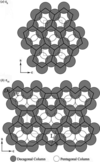Figure 8 The construction of ∊6 and ∊16 from the decagonal columns (Dc) and pentagonal columns (Pc) running along the b axis. The structure of ∊6 is constructed by only one type of hexagonal tiles (outlined) built from six decagonal columns with two intersecting pentagonal columns inside. The structure of ∊16 is constructed by two types of tiles alternating along the a and c axes (outlined): a banana-shaped tile and a pentagonal tile.

We expect that it will be possible to obtain the structures of the complete series and describe them using the stacks of decagonal and pentagonal columns. Furthermore, a detailed structure model of the decagonal quasicrystal (d-Al–TM) can possibly be deduced from these common features.

### 4. Conclusion

A series of approximants of quasicrystals are expected to be built from similar atomic clusters, resulting in similar intensity distributions of reflections in reciprocal space. The similarity of the intensity distributions leads to similar structure-factor amplitudes and phases of the related reflections, especially for the strongest reflections. Based on this knowledge, the structure-factor amplitudes and phases of an unknown structure can be deduced from those of a related known structure. A structure model can be deduced from the three-dimensional electron-density map obtained by inverse Fourier transformation of the structure factors. Such a case was demonstrated here successfully on the complex and unknown structure of 16 based on the related known structure of 6. The derived structure model agrees well with the experimental precession electron-diffraction patterns and high-resolution transmission electron microscopy images. The structure of 16 with 153 unique atoms within the unit cell is the most complex quasicrystal approximant predicted or solved so far. The strong-reflections approach has once again proven to be an effective method for predicting and solving unknown quasicrystal approximants.

### Footnotes

1Supplementary data for this paper are available from the IUCr electronic archives (Reference: DR5024). Services for accessing these data are described at the back of the journal.

### Acknowledgements

This study was supported by the Swedish Research Council (VR) and the Göran-Gustafsson Foundation.

### References

This is an open-access article distributed under the terms of the Creative Commons Attribution (CC-BY) Licence, which permits unrestricted use, distribution, and reproduction in any medium, provided the original authors and source are cited.STRUCTURAL SCIENCECRYSTAL ENGINEERINGMATERIALS
ISSN: 2052-5206